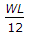# Civil Engineering - RCC Structures Design

### Exercise :: RCC Structures Design - Section 3

1.

If permissible working stresses in steel and concrete are respectively 1400 kg/cm2 and 80 kg/cm2 and modular ratio is 18, in a beam reinforced in tension side and of width 30 cm and having effective depth 46 cm, the lever arms of the section, is

 A. 37 cm B. 38 cm C. 39 cm D. 40 cm

Explanation:

No answer description available for this question. Let us discuss.

2.

If the maximum bending moment of a simply supported slab is M Kg.cm, the effective depth of the slab is (where Q is M.R. factor)

 A.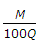B.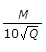C.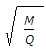D.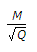E.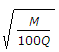Explanation:

No answer description available for this question. Let us discuss.

3.

Though the effective depth of a T-beam is the distance between the top compression edge to the centre of the tensile reinforcement, for heavy loads, it is taken as

 A.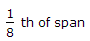B.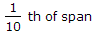C.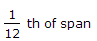D.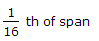E.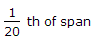Explanation:

No answer description available for this question. Let us discuss.

4.

Design of R.C.C. cantilever beams, is based on the resultant force at

 A. fixed end B. free end C. mid span D. mid span and fixed support.

Explanation:

No answer description available for this question. Let us discuss.

5.

If the length of a wall on either side of a lintel opening is at least half of its effective span L, the load W carried by the lintel is equivalent to the weight of brickwork contained in an equilateral triangle, producing a maximum bending moment

 A.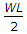B.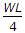C.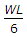D.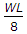E.